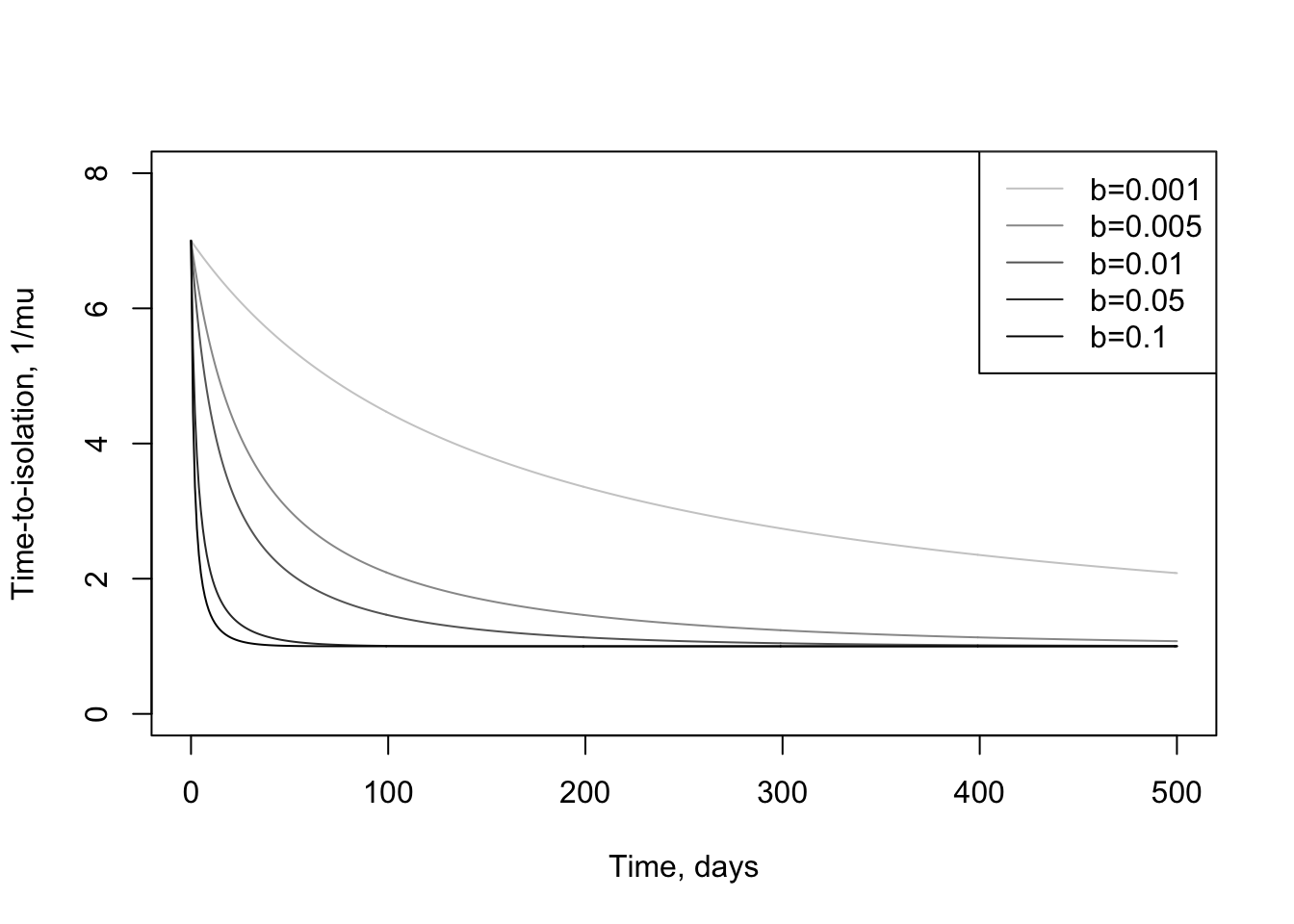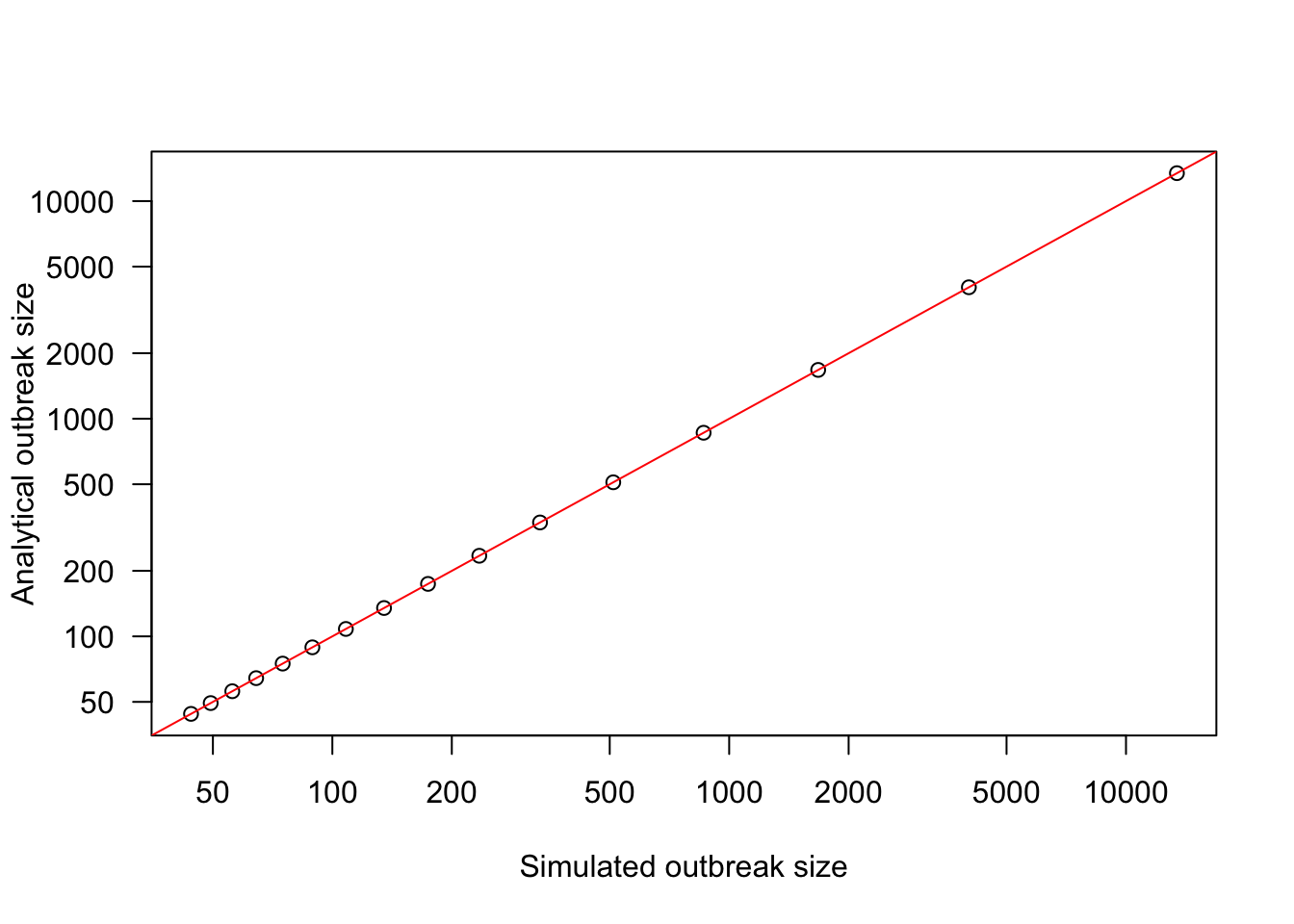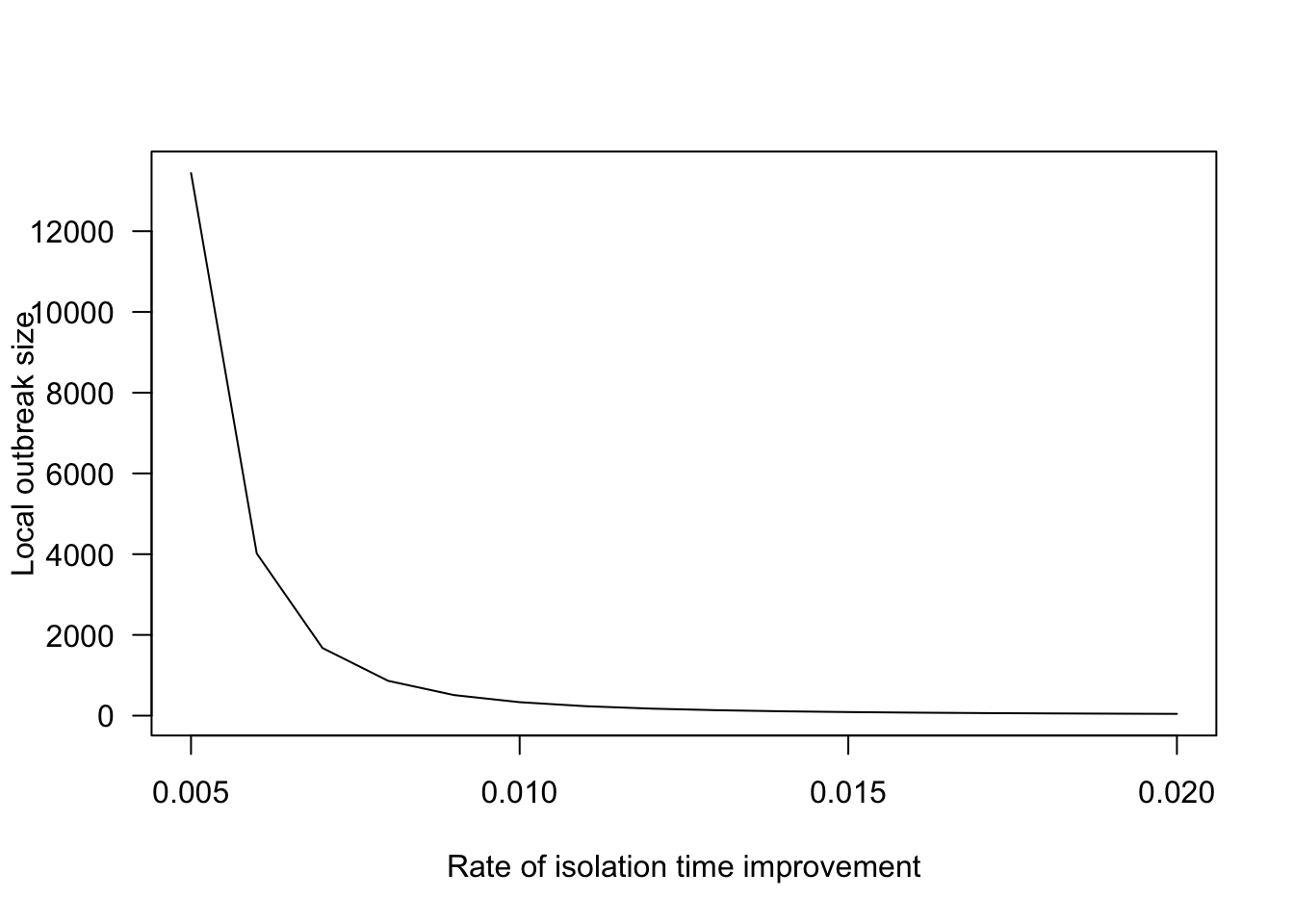## Overview

In order to estimate the potential size of the ongoing COVID-19 outbreak, we adapt a model previously developed for the recent Ebola outbreak (Drake and Park 2016). The model assumes that local transmission is contained by increasing the rate of patient isolation over time (as experience, diagnostics and capacity are augmented) effectively shortening the infectious period such that transmission is halted. These dynamics may be modeled as a special case of the generalized birth-death model (Kendall 1948). Embedding this in a global model, via probabilistic sparking of new outbreaks elsewhere, the full model is able to generate a distribution of global epidemic sizes using relatively few, but estimable parameters, and is useful in providing best-case, worst-case and average-case scenarios when region-specific data to support bespoke models is still in collection/collation phase. The embedding of the local transmission model in a global probabilistic framework is done in exactly the same way as previously described (Drake and Park 2016). However, formerly the local outbreak dynamics in the Ebola model were assumed to be curtailed by reduced force of infection, whereas here it is by decreased time-to-isolation.

## Local transmission and mean outbreak size

We consider a birth-death process where births (infections) occur at rate $$\lambda$$ and deaths (death, recovery or isolation) occur at a rate $$\mu=a_1-(a_1-a_0)e^{-bt}$$. Following pioneering work on the generalized birth-death model (Kendall 1948) the mean final size is given by

$M=1+\frac{\lambda e^f}{b f}\left(\frac{1}{f}\right)^{\frac{g}{b}-1} \gamma \left( \frac{g}{b}, f \right)$

where $$\gamma$$ is the lower incomplete gamma function, $$f=\frac{a_1-a_0}{b}$$ and $$g=a_1-\lambda$$.

The learning rate, $$b$$, then, describes how quickly local time-to-isolation of a patient improves. For this scenario, we assume improvement is from 7 days infectious in a community (an assumed natural infectious period), to a minimum of one day:Figure 1: Example parameterizations of how patient time-to-isolation decreases during a local outbreak as a function of learning rate $$b$$.

To satisfy ourselves that the result for mean local outbreak size is correct, we can measure the final size from a deterministic model and compare it to the analytical result.Figure 2: Comparison of outbreak sizes from deterministic simulation of the birth-death model and the analytical result presented, with all comparisons lying on the the 1:1 line.

Further, we can see how local outbreak size drops as a function of rate of improving patient isolation ($$b$$), done here using other parameters $$a_1=1,a_0=1/7,\lambda=0.371$$. Parameters $$a_0$$ and $$\lambda$$ are estimated from data (CEID and others). Together, parameters $$a_0$$ and $$a_1$$ describe a situation where patient isolation during local outbreaks is initially absent (leading to a recovery rate of 1/7 days$$^{-1}$$) and improves (to one day) with parameter $$b$$ describing how fast a local area moves between these two isolation times. Parameters $$\lambda$$ is in broad agreement with estimates. Parameters describing recovery rate and augmented patient isolation are plausible estimates.Figure 3: Local outbreak size as a function of learning rate $$b$$, here varied between $$b=0.005$$ and $$b=0.02$$, with $$a_1=1,a_0=1/7,\lambda=0.371$$.

## Scaling from local to global case number estimates

To scale up from local outbreaks to epidemics we adopt a probabilistic model in which local outbreaks are connected by movement of infected individuals among communities. In general, we assume that the number of uninfected communities is large so that the chance that an infected individual sparks an outbreak in another community may be represented by a small constant $$0 < \varepsilon \ll 1$$. Let $$p_x$$ be the probability mass function for an outbreak of size $$x$$. Since the probability that an individual doesn’t spark a secondary outbreak is $$1-\varepsilon$$, the probability that an outbreak of size $$x$$ fails to spark a secondary outbreak will be $$(1- \varepsilon)^x$$ by an assumption of independence. The probability that there is an outbreak of size $$x$$ and that it fails to spark any secondary outbreaks is therefore $$p_x(1-\varepsilon)^x$$. By enumeration of all possible outbreak sizes, the probability that an outbreak of unknown size will spark at least one secondary outbreak is

$\label{eq:alpha} \alpha = 1- \sum_{x=1}^\infty p_x(1-\varepsilon)^x.$

Using mathematical analysis of distributions we arrive at an expected (mean) value for the total epidemic size to be

$\label{eq:mean} 1/\pi = M+(M+1)(\frac{1}{1-\alpha}) - \frac{\alpha}{(1-\alpha)}.$

This derivation of this equation relies on approximations for the probability of a secondary outbreak given an outbreak of unknown size and the distribution of outbreak sizes (assumed to be approximated by a geometric distribution), as well as the assumption that outbreak number and outbreak sizes are independent. By embedding the local mean outbreak size estimate in a model that assumes each infectious person has a probability of sparking a new outbreak, we arrive at a distribution of global outbreak sizes, as a function of just a few model parameters. From this distribution, we illusrate here the mean, along with best and worst case numbers (1st and 99th percentile, respectively) for global outbreak size with $$\lambda=0.371,a_0=1/7,a_1=1$$ as a function of sparking probability ($$\varepsilon$$) and learning rate ($$b$$).